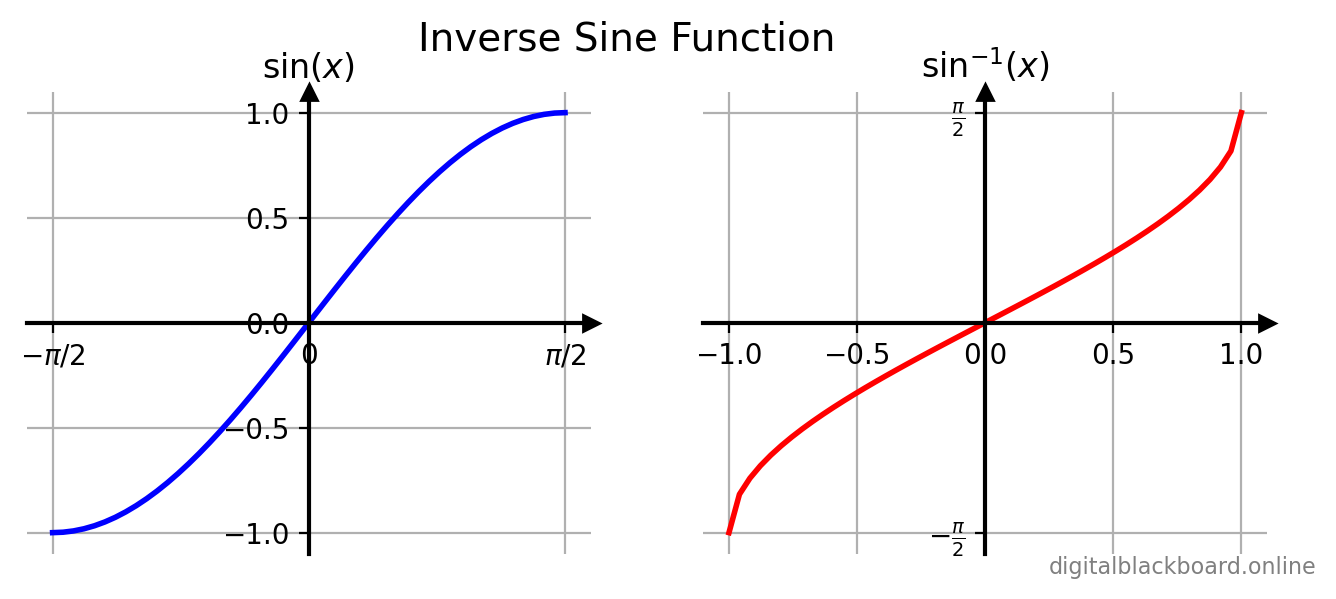## Introduction

In this article, we will examine the inverses of the 3 basic trigonometric ufuncs:

Function Ufunc Input x Domain of Function Description
$\sin^{-1} x$ np.arcsin(x) real $-1 \leq x \leq 1$ Inverse Sine function
$\cos^{-1} x$ np.arccos(x) real $-1 \leq x \leq 1$ Inverse Cosine function
$\tan^{-1} x$ np.arctan(x) real $-\infty \leq x \leq \infty$ Inverse Tangent function

## The Inverse Sine Function

The inverse sine function is defined only when we restrict the domain of $\sin x$ to $[-\frac{\pi}{2},\frac{\pi}{2}]$ so that $\sin x$ is one-to-one. The inverse sine function is defined as follows:

Definition

$y= \sin^{-1}x \iff x = \sin y$

where $-1 \leq x \leq 1$ and $-\frac{\pi}{2} \leq y \leq \frac{\pi}{2}$

The $\sin x$ function and its inverse $\sin^{-1}x$ are plotted side by side in the following figure.The inverse sine function can be computed using the NumPy ufunc np.arcsin(x).

Example

Computing the $\sin^{-1}x$ function.

1import numpy as np
2x = np.linspace(-1, 1, 9)
3print(x) # x values
4print(np.around(np.arccos(x),3)) # arcsin values

[-1.   -0.75 -0.5  -0.25  0.    0.25  0.5   0.75  1.  ]

[-1.571 -0.848 -0.524 -0.253  0.     0.253  0.524  0.848  1.571]

Caution

The superscript $-1$ that appears in $y = \sin^{-1}x$ is not an exponent but the symbol used to denote the inverse function $f^{-1}$ of $f$. To avoid confusion, the notation $y = \text{Arcsin}~x$ may sometimes be used instead of $y = \sin^{-1}x$.

## The Inverse Cosine Function

The inverse cosine function is defined only when we restrict the domain of $\cos x$ to $[0,\pi]$ so that $\cos x$ is one-to-one. The inverse sine function is defined as follows:

Definition

$y= \cos^{-1}x \iff x = \cos y$

where $-1 \leq x \leq 1$ and $0 \leq y \leq \pi$

The $\cos x$ function and its inverse $\cos^{-1}x$ are plotted side by side in the following figure.The inverse cosine function can be computed using the NumPy ufunc np.arccos(x).

Example

Computing the $\cos^{-1}x$ function.

1x = np.linspace(-1, 1, 9)
2print(x) # x values
3print(np.around(np.arccos(x),3)) # arccos values

[-1.   -0.75 -0.5  -0.25  0.    0.25  0.5   0.75  1.  ]

[3.142 2.419 2.094 1.823 1.571 1.318 1.047 0.723 0.   ]


## The Inverse Tangent Function

The inverse tangent function is defined only when we restrict the domain of $\tan x$ to $(-\frac{\pi}{2},\frac{\pi}{2})$ so that $\tan x$ is one-to-one. The inverse tangent function $y= \tan^{-1}x$ is defined as follows:

Definition

$y= \tan^{-1}x \iff x = \tan y$

where $-\infty < x < \infty~$ and $~-\frac{\pi}{2} < y < \frac{\pi}{2}$

The $\tan x$ function and its inverse $\tan^{-1}x$ are plotted side by side in the following figure.The inverse tangent function can be computed using the NumPy ufunc np.arctan(x).

Example

Computing the $\tan^{-1}x$ function.

1x = np.linspace(-100, 100, 9)
2print(x) # x values
3print(np.around(np.arctan(x),3)) # arccos values

[-100.  -75.  -50.  -25.    0.   25.   50.   75.  100.]

[-1.561 -1.557 -1.551 -1.531  0.     1.531  1.551  1.557  1.561]